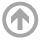Spring 2015 :: Mathematics Department :: USNA
Mathematics Department

# Colloquium Series

## Spring 2015

All talks are from 3:45-4:45 p.m. in the Colloquium Room, unless otherwise specified.

• Apr
23
• A Game of Crowns
Prof. Pam Harris
USMA (West Point)
Time: 03:45 PM

#### View Abstract

The generalized crown, \$\mathbb{S}^k_n\$, is a well-known family of bipartite graphs whose order dimension is given in terms of the parameters \$n\$ and \$k\$. To each generalized crown one can associate a graph of critical pairs whose chromatic number is bounded above by the order dimension of the poset. In this talk we characterize the adjacency matrix of these graphs and through Mathematica can quickly produce an image of these graphs.
• Apr
08
• Interplay between Mathematics and Physics
Shouhong Wang
Indiana University
Time: 03:45 PM

#### View Abstract

In this talk, we shall present two examples, demonstrating the symbiotic interplay between modern physics and advanced mathematics. The first example is on the mystery of dark matter and dark energy phenomena. We show that the presence of dark energy and dark matter gives rise to a new basic principle, the principle of interaction dynamics (PID). Intuitively, PID takes the variation under the energy-momentum constraints. With PID, we derive a new set of gravitational field equations, which demonstrate that gravity can display both attractive and repulsive effect. Dark energy and dark matter are then simply a property of gravity. This part of the talk is based on the joint work with Tian Ma. The second example introduces a novel approach to deal with the parameterization problem of the "small" spatial scales by the "large" ones for stochastic partial differential equations. This approach relies on stochastic parameterizing manifolds (PMs) which are random manifolds aiming to provide---in a mean square sense---approximate parameterizations of the small scales by the large ones. Backward-forward systems will be introduced to give access to such PMs as pullback limits depending---through the nonlinear terms---on the time-history of the dynamics of the low modes. It will be shown that the corresponding pullback limits can be efficiently determined in practice, leading in turn to an operational procedure for the derivation of non-Markovian reduced equations able to achieve good modeling performances. A stochastic Burgers-type equation will serve to illustrate how the memory effects conveyed by such reduced systems play a key role to reach such performances, in particular concerning the ability of these reduced systems to capture transitions or large excursions caused by the interactions between the noise and the nonlinear term. This part of the talk is based on a joint work with Mickael Chekroun and Honghu Liu.
• Apr
07
• Fast Times in Linear Programming: Early Success, Two Revolutions, and Continuing Mysteries
Prof. Margaret Wright
New York University
Time: 07:30 PM

#### View Abstract

Linear programming (LP), which isn't really about programming, is a simple-to-state mathematical problem of enormous practical importance. The dramatic saga of LP solution methods began immediately after World War II with unexpected practical success that continued for more than 30 years despite theoretical reservations; next came two sweeping revolutions whose effects are often misunderstood. This talk will describe mathematical and computational issues from the history of LP, enlivened by controversy and international politics, as well as some remaining mysteries.
• Apr
01
• Tools from Computational Topology, with applications in the life sciences
Prof. Sarah Day
College of William and Mary
Time: 03:45 PM

#### View Abstract

The field of topology, and in particular computational topology, has produced a powerful set of tools for studying both model systems and data measured directly from physical systems. I will focus on three classes of topological tools: computational homology, topological persistence, and, very briefly, Conley index theory. To illustrate their use, I will discuss recent projects studying coupled-patch population dynamics, flickering red blood cells, and pulse-coupled neurons.
• Mar
04
• Strong shift equivalence and algebraic K-theory
Prof. Mike Boyle
University of Maryland
Time: 03:45 PM

#### View Abstract

This will be a colloquium talk, assuming no background in K-theory. Let R (always assumed to contain 0 and 1) be a subset of a ring. Let A,B be square matrices over R (not necessarily of equal size). A and B over R are elementary strong shift equivalent over R (ESSE-R) if there exist matrices U,V over R such that A=UV and B=VU. A and B are strong shift equivalent over R (SSE-R) if they are connected by a chain of elementary strong shift equivalences. If R is a ring, what does it mean for A and B to be strong shift equivalent? This question is motivated by classification problems in symbolic dynamics, as I'll describe, but is natural enough on its own. In his 1973 Annals paper, Williams took the first step, introducing shift equivalence (SE) as an invariant of strong shift equivalence. Whether SE implies SSE for a ring R remained open. It turns out that for a ring R, the refinement of SE by SSE can be nontrivial, and is captured exactly by the algebraic K-theory group NK_1(R). This is joint work with Scott Schmieding.
• Jan
21
• Special loci for the moduli space of rational maps
LT Brian Stout
USNA
Time: 03:45 PM

#### ARCHIVES